# Test: Electronic Devices & Digital Communications - 1

## 15 Questions MCQ Test GATE ECE (Electronics) 2023 Mock Test Series | Test: Electronic Devices & Digital Communications - 1

Description
Attempt Test: Electronic Devices & Digital Communications - 1 | 15 questions in 45 minutes | Mock test for Electronics and Communication Engineering (ECE) preparation | Free important questions MCQ to study GATE ECE (Electronics) 2023 Mock Test Series for Electronics and Communication Engineering (ECE) Exam | Download free PDF with solutions
QUESTION: 1

### Concentration of free electrons in a copper wire (diameter=1.03 mm) is 8.4 x 1028 electrons/m3. If the current in the wire is 2A, then drift velocity is

Solution: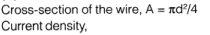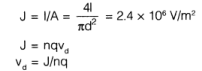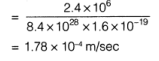QUESTION: 2

### If a donor-type impurity is added to the extent of 1 atom per 108 silicon atoms, find the resistivity.

Solution: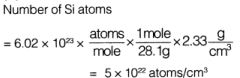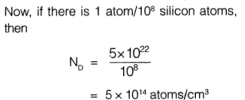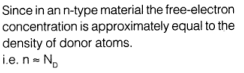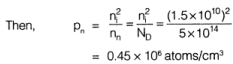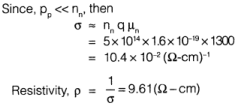QUESTION: 3

### The p-type substrate in a monolithic circuit should be connected to

Solution:
QUESTION: 4

What is the ratio of current for a forward bias of 0.05 V to the current for the same magnitude of reverse bias?

Solution: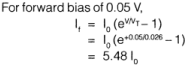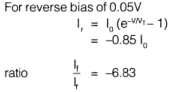QUESTION: 5

The probability of an electron being thermally promoted to the conduction band in Si(∈g=1.107 eV) at room temperature (25°C)?

Solution:
QUESTION: 6

One curve of an n-channel MOSFET is characterized by the following parameters
ID(sat) = 0.2 mA
VDs = 0.8 V

VTN =0.8V

The value of conduction parameter is

Solution: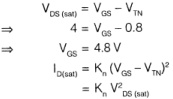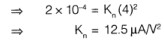QUESTION: 7

Sampling rate needed to achieve (SNR)0/P ≥ 45 dB with fs = 10 MHz in a PCM system

Solution: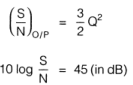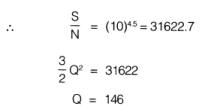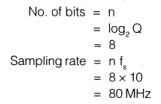QUESTION: 8

In a 64 step binary PCM system with a reference scale from 0 to 6.4 volts, the RMS quantization error will be,

Solution: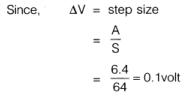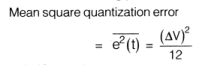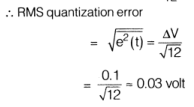QUESTION: 9

In a BPSK signal detector, the local oscillator has a fixed phase error of 25.2°. Reduction in SNR will be by a factor of _____________

Solution: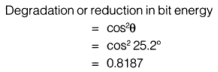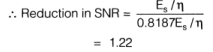QUESTION: 10

The SQR (Signal to Quantization Noise Ratio) of a system is 25.8 dB for 16 quantization levels. The percentage increase in SQR for 128 quantization levels is

Solution: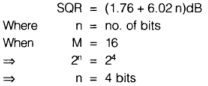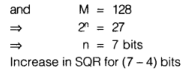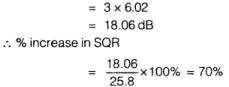QUESTION: 11

Error distance in 8 QAM modulation scheme is

Solution: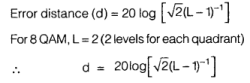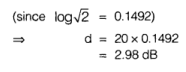QUESTION: 12

A system with transfer function H(s) is driven by a white noise x(t) with power density N0. The Auto-correlation of the output signal is measured as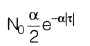where α > 0. A possible transfer function H(s) for system wilt be

Solution: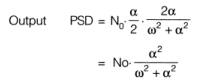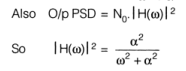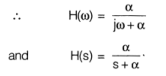QUESTION: 13

A vestigial SSB system is used to modulate a TV signal having bandwidth equal to 4 MHz. If roll-off factor is 1, the bandwidth required to transmit the signal is given by _________ MHz

Solution:
QUESTION: 14

The capacitance of varactor varies from 5 to 50 pF. Two such varactor diodes are used in the tunning circuit shown in figure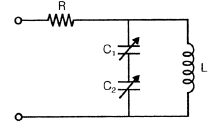If L= 10 mH, the tuning range of the circuit

Solution: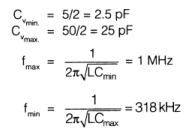QUESTION: 15

The variation of drain current with gate to source voltage (lD - VGS characteristic) of a MOSFET is shown in figure. The MOSFET is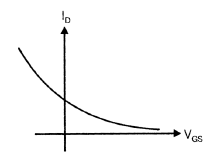Solution:Use Code STAYHOME200 and get INR 200 additional OFF Use Coupon Code